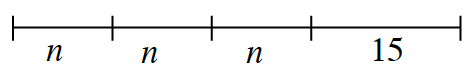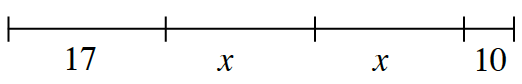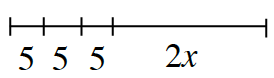### Home > CC1MN > Chapter 6 > Lesson 6.3.4 > Problem6-104

6-104.

Write an algebraic expression to represent the length of each segment shown below.

1.$n + n + n + 15 or 3n + 15$

2.$17 + x + x + 10$. Can you find a simpler
way to write this expression?

3.• See parts (a) and (b).